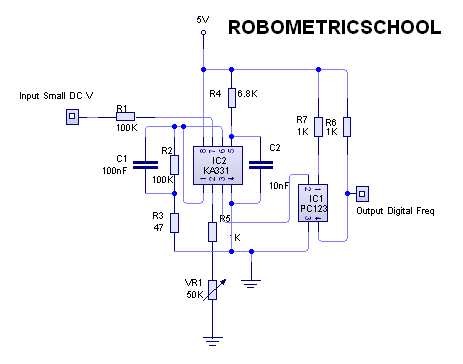Sunday, January 20, 2013

Electronic Circuit Schematic Analog to Frequency Converter using KA331 and Optocoupler PC123

Robometricschool.- The next electronic circuit diagram that i will show to you is Analog to Frequency Converter (AFC) using KA331 and optocoupler PC123 as alternative of Analog to Digital Converter ADC.

Like commonly that if you want to change analog signal to digital signal usually use ADC or Analog to Digital Converter to convert analog signal into digital signal and then you can processed this signal using microcontroller to do another form.

In here i want to give you special circuit schematic of analog to frequency converter using KA331 and also optocoupler PC 123 like in figure 1. bellow:Figure 1. Analog to Frequency Converter using KA331 and PC123 Drawing use PCB Wizard software by Robometricschool

According electronic circuit schematic above, we use IC KA331 to convert analog signal to frequency signal. From KA331 datasheet we know that KA331 is analog voltage to frequency converter provides the output pulse train at a frequency precisely proportional to the applied input voltage. The KA331 also can operate at power supplies as low as 4.0V and be changed output frequency from 1Hz to 100KHz. It is ideally suited for use in simple low-cost circuit for analog-to digital conversion, long term integration, linear frequency modulation or demodulation, frequency-to-voltage conversion, and many other functions.

Input of circuit schematic of analog voltage to frequency using KA331 and PC123 is DC small analog voltage that will connected in pin 7 as input KA331. Output of this IC is Pulse output compatible with all logic form with wide dynamic range: 100dB min at 10KHz full scale frequency. We can adjust output signal using VR1 50Kohm, if you change VR1 with smaller value so the output range will be narrow than using VR1 50K ohm.

To get good output digital signal frequency we can use PC123 as optocoupler IC that will make input signal more good digital signal and make signal more stability. To know more about IC PC123 you can see PC123 datasheet.

From Electronic Circuit Schematic Analog to Frequency Converter using KA331 and Optocoupler PC123 like in figure 1 above can be used in application like temperature signal condition, frequency counter, etc.

Maybe i will show you next time project that using Analog to Frequency Converter using KA331 and Optocoupler PC123 like in electronic circuit schematic figure 1 above as used to make digital termometer using LM35. please wait.(her)